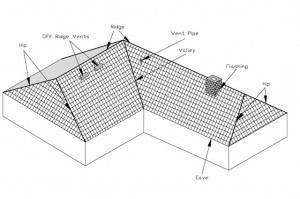•• # Dimensions of a Roof

The roof size will depend on how large the house or building will be.Different house dimensions and styles means there is no standard size. But when estimating roof requirements, some basic principles should be followed.

Roofing materials are always sold in squares. The square is equal to the amount needed to face 100 sq ft on the roof when it is put in place. The manufacturer determines the pieces required to envelop 100 sq ft (called the square of material).

The manufacturers will determine this because roof coverings vary widely. Overlap and layers will have different measurements. In simple terms: 1 square equals 100 sq ft.

### Assessing the Roofing Material Required

The best way to know is to ask the contractor to assess the roof size. This info will be available in the building / housing plans too. Another method is to measure each individual roof surface. Note: do not attempt this if you don’t have any experience in measuring roofs. Ask a roofing contractor to do it for you.

### Measuring from the Ground

This is another, albeit more complex method. Begin by measuring the house outside walls. Multiply the width x length to obtain the house area in sq ft. if your house isn’t square or rectangle, compute each piece then total the sums. Example: a 50 x 30 ft (length x width) = 1500 sq ft.

To get the roof size, convert the sq ft area to roofing squares. Assuming the area is 1500 sq ft, it should be divided by 100 (100 is always the number to use). The answer is 15 squares.

### Calculating the Roof Pitch / Slope

A low pitch usually has a 3:12 pitch. This means the roof goes up 3 ft per 12 ft of the horizontal base. Assuming the pitch doesn’t go beyond 5:12, multiply by 1.15 or 1.25. For the medium roof pitch (6:12 to 9:12), multiply by 1.25 to 1.4 to obtain the roofing squares.

Note: the multiplier depends on the valleys. end walls etc on the roof. To get the roof size for a high pitch (9:12 and above), multiply by 1.41 up to 1.7. Depending on its complexity, a multiplier of 1.5 may be needed.

Presume the roof has a pitch of 6:12 with an end wall and two valleys. Multiply it by 1.35. If the house is 30 x 50 ft (equal to 15 roofing squares), multiply it by 1.35. The total will be 20 roofing squares.

### Warnings

Low pitch roofs can be walked on, but medium and high roofs cannot. Not only are medium and high pitch roofs more difficult to setup; they’re also more costly. The low, medium and high pitch / slope cited above are just examples.

There are many more variants. Also make sure the calculations for the house walls are accurate. Double checking the figures is recommended.

If you know the roof size, you’ll never be duped by contractors into paying for more material than you need. This can spell substantial savings on construction costs.